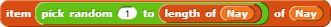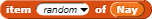Basic Algebra From 1-10
P.S. The code is SOOOOOO complicated.

Im taking algreba 1 how nice

Looks cool! Just a question, why are the equations stored as 2 lists of 5 equations each, instead of 1 list with all 10 equations?

P.S. You can replacewithI'm wondering why you have specific equations built in, rather than create one randomly each time. Choose a random value of a, then choose a multiplier, then choose a value to add, and then the program computes the result of the arithmetic and that's the right hand side.

You're right. I'll try to do that.

Good job !

a³ ÷ 4 = 72
The result of this is not an integer. I answered with 6.6, but said it was wrong.
* 4: a³ = 288
^ (1/3): a = 6.6038545

one thing: hooray is spelled as horay in the project. other than that, cool!

also, please use asteruses (?) (I mean the * symbol) instead of those dots.

I can do that, or I can make this symbol: ×. Which do you prefer?

the ×.

Don't worry, I fixed it. My math skills aren't the best LOL.